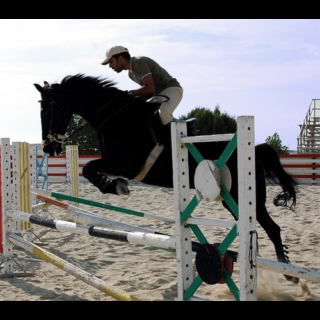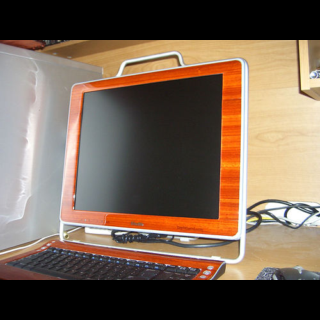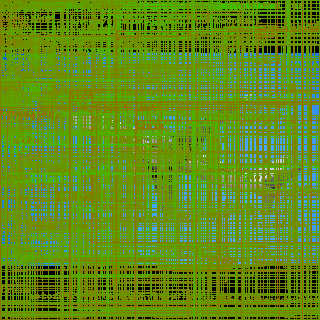# Building Tiny YOLO from scratch using PyTorch

posted on 2020-04-23T12:09:34Z · last modified on 2020-09-01T09:57:11Z · view page on GitHub
tags:

In this series we'll go over YOLO (You Only Look Once), a state-of the art object detection deep neural network. In this blog post, we'll build the simplest YOLO network: Tiny YOLO v2. This stripped down version of YOLO will yield the easiest introduction to the neural network structure of YOLO, while still providing close to state-of-the-art performance.

## Implementing YOLO in PyTorch¶

Why would we want to implement YOLO in PyTorch? Didn't the original author already implement YOLO in some framework?

Well, yes, YOLO is implemented in Darknet, a deep learning framework from the same author as YOLO. And although I have no doubt that this is an excellent framework, it's made for C... I prefer to stick with Python.

However, the specifications for all YOLO networks can be found on GitHub and on the YOLO homepage. This allows us to quite easily implement these networks with a flexible deep learning framework like PyTorch.

## Short introduction to YOLO¶

YOLO is a computer vision deep neural network that is specialized in very fast object detection and recognition.

To circumvent the risk of beating a dead horse, here are some excellent resources that explain what YOLO is, why it's special and why it's better than its competitors for fast object detection:

Here is my relatively short take on it (if it's not immediately clear, it should become more clear once we start implementing it):

YOLO is a deep convolutional neural network that scans an image for objects. The network divides the image into a coarse grid of 32x32 pixel cells. Each cell of the grid predicts a number of object classes with corresponding bounding boxes and a confidence score. The bounding boxes with highest confidence are kept, while the other predicted bounding boxes are ignored.

The number of bounding boxes each cell predicts depends on the number of predefined anchors. In fact, since YOLO v2, each cell, only predicts the offset of the bounding box to the middle of the cell and the offset of the size of the bounding box to each predifined anchor.

This all means that bigger images will allow for more predictions to be made (as more 32x32 cells fit into the image). Additionally, the number of predifined anchors directly will also influence the number of predictions made by each cell, and thus the total number of predictions.

Great! For now, you know everything you need to know! Let's move on!

## PASCAL Visual Object Classes dataset¶

In this part, we will use the PASCAL Visual Object Classes (VOC) dataset. This is a dataset of pictures which are labeled with bounding boxes and corresponding object classes. This dataset contains only 20 quite general classes, which makes it a perfect introductory dataset. Let's download this first. If you try this at home: this takes some time!

In :
import os
import tarfile
import urllib.request

# the VOC object classes.
CLASSES = (
"aeroplane",
"bicycle",
"bird",
"boat",
"bottle",
"bus",
"car",
"cat",
"chair",
"cow",
"diningtable",
"dog",
"horse",
"motorbike",
"person",
"pottedplant",
"sheep",
"sofa",
"train",
"tvmonitor",
)
CLASS2NUM = {class_:idx for idx, class_ in enumerate(CLASSES)}

if not os.path.exists("VOCdevkit"):
if not os.path.exists("VOC.tar"):
urllib.request.urlretrieve(
"https://pjreddie.com/media/files/VOCtrainval_11-May-2012.tar", "VOC.tar"
)
with tarfile.open("VOC.tar") as tar:
tar.extractall()


Now that we downloaded the VOC data, let's create a function to load the data. The following function will load a single image as a PyTorch tensor. Optionally, the image can be resized to a given size by scaling and padding.

In :
import torchvision
from PIL import Image

filename = f"VOCdevkit/VOC2012/JPEGImages/2008_{str(idx).zfill(6)}.jpg"
img = Image.open(filename)
transforms = [torchvision.transforms.ToTensor()]
try:
width, height = size
except TypeError:
width = height = size
scale = min(width / img.width, height / img.height)
new_width, new_height = int(img.width * scale), int(img.height * scale)
diff_width, diff_height = width - new_width, height - new_height
resize = torchvision.transforms.Resize(size=(new_height, new_width))
diff_width // 2,
diff_height // 2,
diff_width // 2 + diff_width % 2,
diff_height // 2 + diff_height % 2,
)
)
transforms = [resize, pad] + transforms
transformation = torchvision.transforms.Compose(transforms)
x = transformation(img).to(device)
return x


The following function will load multiple images in a batch. This function requires a size to be given to resize each image to. Otherwise the PyTorch tensors cannot be stacked in the first dimension (to form a batch).

In :
import torch

imgs = [load_image(idx, size=size, device="cpu") for idx in idxs]
x = torch.stack(imgs, 0)
return x.to(device)


Finally, we suspect it might be useful to be view the images:

In :
from IPython.display import display

def show_images(x):
transform = torchvision.transforms.ToPILImage()
if x.dim() == 3:
display(transform(x))
elif x.dim() == 4:
for xx in x:
display(transform(xx))
else:
raise ValueError


Let's load four images and visualize them:

In :
imgs = load_image_batch([8, 16, 33, 60], size=320)
show_images(imgs)## Tiny YOLO v2 specification¶

Tiny YOLO consists of several convolutional layers with leaky ReLU activation. This activation is similar to a normal ReLU activation, but values smaller than zero are multiplied by 0.1 in stead of 0.

Each of those layers extracts features from the image, followed by pooling layers that downscale the image. In total, there will be 5 pooling layers of size 2 and stride 2, which explains the intuitive notion of a 32x32 grid cell responsible for each prediction as the image is scaled down by a factor of 2⁵=32.

This means that after the complete convolutial stack, the image of width W and height H is transformed to a tensor of width w=W/32 and height h=H/32. This Convolutional transformation thus transforms an image of W*H pixels into a tensor of w*h cells, each of which can be related to the overlayed grid of 32x32 pixel cells.

Each of these cells will now predict a width and height ofsset (dw, dh) to the 5 predifined Tiny YOLO anchors: (1.08,1.19), (3.42,4.41), (6.63,11.38), (9.42,5.11), (16.62,10.52). For each of the resulting bounding boxes, an offset (dx, dy) to the center (x0, y0) of the cell is also predicted along with a confidence of the bounding box C.

Assuming we follow the Tiny YOLO VOC specification, we expect the Network to select 1 of 20 classes to predict for each bounding box. This means the network will predict a (softmax) probability for each of the 20 classes to predict on top of the 5 features related to the bounding box itself. This means that the complete convolutional stack will have to transform the image with 3 input channels to a tensor with width w and height h and depth 125: 5 bounding box features together with 20 class probabilites for each of the 5 different predifined anchors.

So this is Tiny YOLO v2 in table form:

#   ImgSize   Layer   Filters   Size   Stride   Pad   Activation
0 320 Convolutional 16 3 1 1 leaky ReLU
1 320 MaxPool - 2 2 0 -
2 160 Convolutional 32 3 1 1 leaky ReLU
3 160 MaxPool - 2 2 0 -
4 80 Convolutional 64 3 1 1 leaky ReLU
5 80 MaxPool - 2 2 0 -
6 40 Convolutional 128 3 1 1 leaky ReLU
7 40 MaxPool - 2 2 0 -
8 20 Convolutional 256 3 1 1 leaky ReLU
9 20 MaxPool - 2 2 0 -
10 10 Convolutional 512 3 1 1 leaky ReLU
11 10 MaxPool - 2 1 1 -
12 10 Convolutional 1024 3 1 1 leaky ReLU
13 10 Convolutional 1024 3 1 1 leaky ReLU
14 10 Convolutional 125 1 1 1 -
13 10 Yolo - - - - -

## Let's build this network in PyTorch, shall we?¶

In :
import torch

class TinyYOLOv2(torch.nn.Module):
def __init__(
self,
num_classes=20,
anchors=(
(1.08, 1.19),
(3.42, 4.41),
(6.63, 11.38),
(9.42, 5.11),
(16.62, 10.52),
),
):
super().__init__()

# Parameters
self.register_buffer("anchors", torch.tensor(anchors))
self.num_classes = num_classes

# Layers
self.relu = torch.nn.LeakyReLU(0.1, inplace=True)
self.pool = torch.nn.MaxPool2d(2, 2)
self.slowpool = torch.nn.MaxPool2d(2, 1)
self.norm1 = torch.nn.BatchNorm2d(16, momentum=0.1)
self.conv1 = torch.nn.Conv2d(3, 16, 3, 1, 1, bias=False)
self.norm2 = torch.nn.BatchNorm2d(32, momentum=0.1)
self.conv2 = torch.nn.Conv2d(16, 32, 3, 1, 1, bias=False)
self.norm3 = torch.nn.BatchNorm2d(64, momentum=0.1)
self.conv3 = torch.nn.Conv2d(32, 64, 3, 1, 1, bias=False)
self.norm4 = torch.nn.BatchNorm2d(128, momentum=0.1)
self.conv4 = torch.nn.Conv2d(64, 128, 3, 1, 1, bias=False)
self.norm5 = torch.nn.BatchNorm2d(256, momentum=0.1)
self.conv5 = torch.nn.Conv2d(128, 256, 3, 1, 1, bias=False)
self.norm6 = torch.nn.BatchNorm2d(512, momentum=0.1)
self.conv6 = torch.nn.Conv2d(256, 512, 3, 1, 1, bias=False)
self.norm7 = torch.nn.BatchNorm2d(1024, momentum=0.1)
self.conv7 = torch.nn.Conv2d(512, 1024, 3, 1, 1, bias=False)
self.norm8 = torch.nn.BatchNorm2d(1024, momentum=0.1)
self.conv8 = torch.nn.Conv2d(1024, 1024, 3, 1, 1, bias=False)
self.conv9 = torch.nn.Conv2d(1024, len(anchors) * (5 + num_classes), 1, 1, 0)

def forward(self, x, yolo=True):
x = self.relu(self.pool(self.norm1(self.conv1(x))))
x = self.relu(self.pool(self.norm2(self.conv2(x))))
x = self.relu(self.pool(self.norm3(self.conv3(x))))
x = self.relu(self.pool(self.norm4(self.conv4(x))))
x = self.relu(self.pool(self.norm5(self.conv5(x))))
x = self.relu(self.norm7(self.conv7(x)))
x = self.relu(self.norm8(self.conv8(x)))
x = self.conv9(x)
if yolo:
x = self.yolo(x)
return x

def yolo(self, x):
# store the original shape of x
nB, _, nH, nW = x.shape

# reshape the x-tensor: (batch size, # anchors, height, width, 5+num_classes)
x = x.view(nB, self.anchors.shape, -1, nH, nW).permute(0, 1, 3, 4, 2)

# get normalized auxiliary tensors
anchors = self.anchors.to(dtype=x.dtype, device=x.device)
range_y, range_x = torch.meshgrid(
torch.arange(nH, dtype=x.dtype, device=x.device),
torch.arange(nW, dtype=x.dtype, device=x.device),
)
anchor_x, anchor_y = anchors[:, 0], anchors[:, 1]

# compute boxes.
x = torch.cat([
(x[:, :, :, :, 0:1].sigmoid() + range_x[None,None,:,:,None]) / nW,  # X center
(x[:, :, :, :, 1:2].sigmoid() + range_y[None,None,:,:,None]) / nH,  # Y center
(x[:, :, :, :, 2:3].exp() * anchor_x[None,:,None,None,None]) / nW,  # Width
(x[:, :, :, :, 3:4].exp() * anchor_y[None,:,None,None,None]) / nH,  # Height
x[:, :, :, :, 4:5].sigmoid(), # confidence
x[:, :, :, :, 5:].softmax(-1), # classes
], -1)

return x # (batch_size, # anchors, height, width, 5+num_classes)


## First prediction of an untrained network¶

Let's see what would happen if we use Tiny YOLO on an image of an airplane:

In :
network = TinyYOLOv2()
input_tensor = load_image_batch(, 320) # batch with single image of an airplane
show_images(input_tensor)
output_tensor = network(input_tensor)
print(output_tensor.shape)torch.Size([1, 5, 10, 10, 25])


As you can see, the result of the propagation of the image through the network is a 1x5x10x10x25 tensor: 1 single element in the batch, 5 anchors, 10x10 cells and 25 features predicted per cell and per anchor.

The first 5 features of those 25 features in the last dimension describe the bounding boxes, while the next 20 features describe the (softmax) probability for the bounding box containing each of the 20 possible classes.

We can try to visualize the bounding boxes predicted:

In :
from PIL import ImageDraw

def show_images_with_boxes(input_tensor, output_tensor):
to_img = torchvision.transforms.ToPILImage()
for img, predictions in zip(input_tensor, output_tensor):
img = to_img(img)
if 0 in predictions.shape: # empty tensor
display(img)
continue
confidences = predictions[..., 4].flatten()
boxes = (
predictions[..., :4].contiguous().view(-1, 4)
)  # only take first four features: x0, y0, w, h
classes = predictions[..., 5:].contiguous().view(boxes.shape, -1)
boxes[:, ::2] *= img.width
boxes[:, 1::2] *= img.height
boxes = (torch.stack([
boxes[:, 0] - boxes[:, 2] / 2,
boxes[:, 1] - boxes[:, 3] / 2,
boxes[:, 0] + boxes[:, 2] / 2,
boxes[:, 1] + boxes[:, 3] / 2,
], -1, ).cpu().to(torch.int32).numpy())
for box, confidence, class_ in zip(boxes, confidences, classes):
if confidence < 0.01:
continue # don't show boxes with very low confidence
# make sure the box fits within the picture:
box = [
max(0, int(box)),
max(0, int(box)),
min(img.width - 1, int(box)),
min(img.height - 1, int(box)),
]
try:  # either the class is given as the sixth feature
idx = int(class_.item())
except ValueError:  # or the 20 softmax probabilities are given as features 6-25
idx = int(torch.max(class_, 0).item())
try:
class_ = CLASSES[idx]  # the first index of torch.max is the argmax.
except IndexError: # if the class index does not exist, don't draw anything:
continue

color = (  # green color when confident, red color when not confident.
int((1 - (confidence.item())**0.8 ) * 255),
int((confidence.item())**0.8 * 255),
0,
)
draw = ImageDraw.Draw(img)
draw.rectangle(box, outline=color)
draw.text(box[:2], class_, fill=color)

display(img)

In :
show_images_with_boxes(input_tensor, output_tensor)Unfortunately, right now, the network does not make any sensible predictions yet, as it is not yet trained. For now, we can skip the training and load the pre-trained weights into the network. Training the YOLO network will be handled in the next part of this series.

Pretrained weights for the Tiny YOLO v2 network trained on the VOC dataset can be downloaded from the darknet website (again, if you're trying this at home: this takes some time to download):

In :
if not os.path.exists("yolov2-tiny-voc.weights"):
urllib.request.urlretrieve(
"https://pjreddie.com/media/files/yolov2-tiny-voc.weights",
"yolov2-tiny-voc.weights",
)


This is just a binary file, with all the weights for each layer stored in sequence. The first three integers in this binary file denote the version of the weight file and the next integer denotes the number of images the network has seen so far to obtain these weights. The following numbers are all of type float32 and denote all the weights in all the layers. Let's create a function to load the weights:

In :
import numpy as np

with open(filename, "rb") as file:
version = np.fromfile(file, count=3, dtype=np.int32)
seen_so_far = np.fromfile(file, count=1, dtype=np.int32)
weights = np.fromfile(file, dtype=np.float32)
idx = 0
for layer in network.children():
if isinstance(layer, torch.nn.Conv2d):
if layer.bias is not None:
n = layer.bias.numel()
layer.bias.data[:] = torch.from_numpy(weights[idx : idx + n]).view_as(layer.bias.data)
idx += n
n = layer.weight.numel()
layer.weight.data[:] = torch.from_numpy(weights[idx : idx + n]).view_as(layer.weight.data)
idx += n
if isinstance(layer, torch.nn.BatchNorm2d):
n = layer.bias.numel()
layer.bias.data[:] = torch.from_numpy(weights[idx : idx + n]).view_as(layer.bias.data)
idx += n
layer.weight.data[:] = torch.from_numpy(weights[idx : idx + n]).view_as(layer.weight.data)
idx += n
layer.running_mean.data[:] = torch.from_numpy(weights[idx : idx + n]).view_as(layer.running_mean)
idx += n
layer.running_var.data[:] = torch.from_numpy(weights[idx : idx + n]).view_as(layer.running_var)
idx += n
if isinstance(layer, torch.nn.Linear):
n = layer.bias.numel()
layer.bias.data[:] = torch.from_numpy(weights[idx : idx + n]).view_as(layer.bias.data)
idx += n
n = layer.weight.numel()
layer.weight.data[:] = torch.from_numpy(weights[idx : idx + n]).view_as(layer.weight.data)
idx += n


In :
load_weights(network)


## Retrieve the bounding boxes from a prediction¶

Remember the image of the airplane, we received a (1, 5, 13, 13, 25) sized tensor with predictions from our network. We still have to translate those to bounding boxes.

In :
input_tensor = load_image_batch(, 320)  # batch with single image of an airplane
output_tensor = network(input_tensor)
show_images_with_boxes(input_tensor, output_tensor)